# Unit conversion - math problems

#### Number of problems found: 868

• The Nürburgring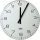The Nürburgring in Germany is a racetrack that hosts racing events all year. Two of the fastest laps ever driven on the track are 6 minutes 47.30 seconds and 6 minutes 52.01 seconds. How much faster is the 6 minutes 47.30 seconds time than the 6 minutes 5
• Temperatures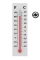Temperatures in degrees Fahrenheit and Celsius are related by the rule F = 1.8C + 32. What is the temperature in degrees Celsius if it is 50°F?
• Gas priceIf a cm3 of gas costs rm 1.50, how many cents would a liter of gas cost? 1 rm = 1 Malaysian Ringgit = 100 Malaysian Ringgit cents = equals 0.21 Euro in 2021/Q3
• I-beam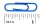If an I-beam is 12’- 6  1/2” long, and it needs to be cut into 4 equal sections, how long will each section be?
• Central Park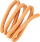Central Park is a rectangular park in New York City and the map scale is 1 cm: 320 m (It means 1 cm on the map represents 320 m in the real world. ). Find the north-south distance if the length on the map is 2.5 cm.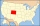The state Colorado has the shape of a rectangle. The map is sold on 2 posters. The first has dimensions of 70 cm and 50 cm in a scale of 1: 1,000,000. The second poster has dimensions of 175 cm and 125 cm. What is the scale of the second poster?
• ThereThere is water in the block-shaped tank with dimensions of 3 m 1.5 m 5 m, it occupies 70% of the tank volume. Find the volume of water in the tank (in hl) .
• A diesel carA diesel car costs \$2599 more than an unleaded petrol car and travels an average of 25,000km per year. Diesel petrol has a consumption rate of 7.4L per 100km and costs \$1.39 per litre. Unleaded petrol has a consumption rate of 8.5L per 100km and costs \$1.
• ParallogramThe Parallelogram base is 24 cm and high 10 cm. How many are such tiles required to cover a floor of area 1080m2?
• Licorice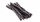A piece of licorice is 2/3 yard long.  If it is cut into 10 equal pieces, how long will each piece of licorice be?
• Cutting wireIf you cut a 3 ½ ft length wire into pieces that are 2 inches long, how many pieces of wire will you have?
• Ferdinand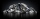Ferdinand went from place A to place B and from there to place C. A is 7.2 km from B and B is 11.7 km from C. Albert went from place A to place D and from there to place C. D is 9.1 km from A and C is 10.8 km from D. Who traveled more and by how much?
• Eights of butter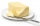How many eights of butter (1/8 of kg = 125 g) can be stored in a box with dimensions of 4 dm, 2 dm, 1.8 dm, if the eighth of butter has dimensions of 8 cm, 5 cm, 3 cm?
• Clock losing time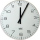A clock is showing 9:30 am. If the clock is 5 minutes slow, what would have been the correct time?
• A straight angle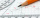A straight angle measures s, so you can subtract or add 85 from What are the measure of their angles?
• Cups of paintI have a board that is 2 meters wide and 3 meters tall. If one cup of paint cover 1,000 square centimeters. How many cups of paints does I need?
• Each ofEach of Suzan's notebooks is 4/5  inches wide. If she has 25 inches of space remaining on her bookshelf, how many notebooks will fit?
• Two citiesOn a map, 1.5 cm represents 50 miles. If the distance between the two cities on the map is 6 cm, how many miles separate the cities?
• Grocery storeYou spend 4/5 of an hour at the mall. You spend 1/3 of that time in the food court. How many minutes are you in the food court?
• Little 2Little fields farm lies on a rectangular plot of land that measures 2 2/4 miles in length and 2 2/3 miles in width. What is it's area in square miles?

Do you have an exciting math question or word problem that you can't solve? Ask a question or post a math problem, and we can try to solve it.

We will send a solution to your e-mail address. Solved examples are also published here. Please enter the e-mail correctly and check whether you don't have a full mailbox.